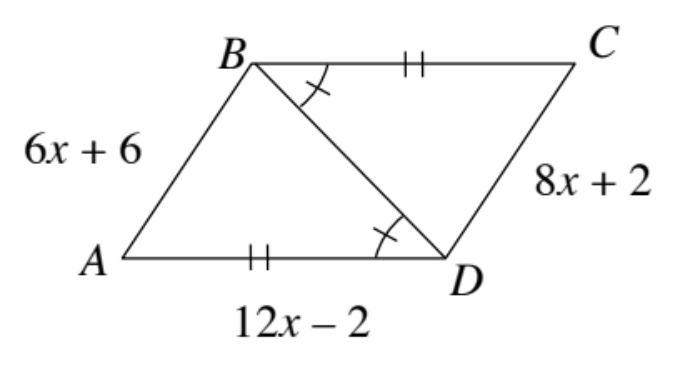### Home > INT2 > Chapter 7 > Lesson 7.1.1 > Problem7-9

7-9.

For the figure at right, determine if the two triangles are congruent. If so, create a flowchart proof to explain why. Then, solve for $x$. If the triangles are not congruent, explain why not.The triangles are congruent. Now show why or how.

Congruent because of SAS$≅$, $x = 2$.